#### Lottery Number Generator

In this Excel tutorial lesson, you will learn how to prepare a lottery number generator sheet in Excel.

## How to generate Random Numbers without Repeats?

Let’s suppose you would like to generate five different random numbers from 1 to 69.

To do that in Column A, let’s use the RAND formula =RAND().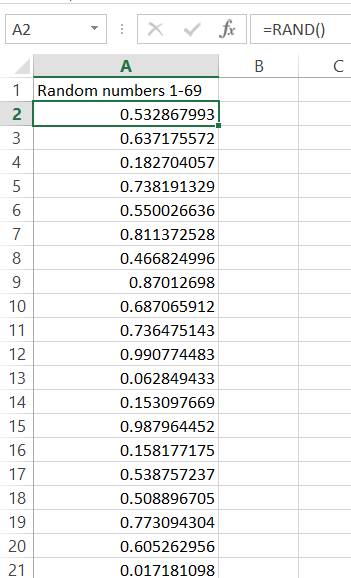Rand Excel function does just one simple thing. It generates a random number. This is exactly what you need here! Let’s scroll down this to have exactly 69 identical cells (you can choose a different number, it’s up to your needs. Lottery Number Generator will work for you. No worries!).

Now the question is.

How to draw the random number from that? You need to create a random number list without repeats.

Imagine the simplicity of the trick. You will generate 69 random numbers, and to pick one, just pick the five rows that generated the largest numbers.

Isn’t it clever?

To pick the first random number, just use this Excel formula: =MATCH(LARGE(\$A\$2:\$A\$70,1),\$A\$2:\$A\$70,0)

It will generate the first random number. If it generated 7, it means that the 7th row generated the largest number. You can check that if you like.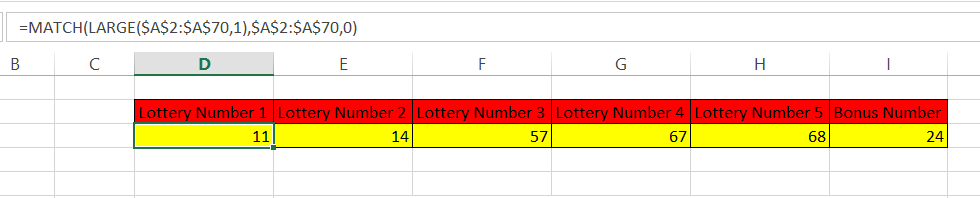To generate more, just change the digit 1 into the next ones (=MATCH(LARGE(\$A\$2:\$A\$70,2),\$A\$2:\$A\$70,0) for second number, =MATCH(LARGE(\$A\$2:\$A\$70,3),\$A\$2:\$A\$70,0) for the third distinct number, and so on).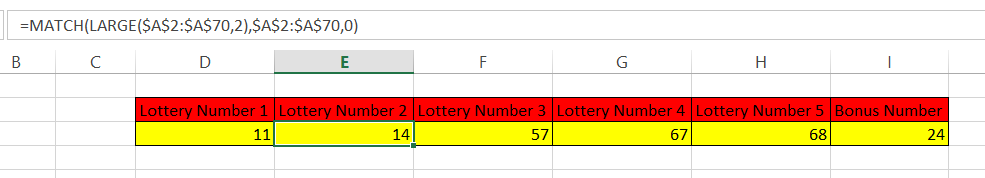### How to generate Random Numbers without Repeats using RANK function?

To generate random numbers between 1 and 69 without repeats in Microsoft Excel, you can also use the following steps:

1. Create a list of numbers from 1 to 69 in a column.
2. Use the “RAND” function to generate a random number between 0 and 1 in a separate column.
3. Use the “RANK” function to rank the numbers based on the random numbers generated in step 2.
4. Use the “INDEX” and “MATCH” functions to retrieve the numbers with the lowest ranks, which will correspond to the random numbers generated in step 2.

Here’s an example of the formula you could use:

=INDEX(\$A\$2:\$A\$69, MATCH(RANK(RAND(),\$B\$2:\$B\$69), RANK(\$B\$2:\$B\$69), 0))

Where A2:A69 is the list of numbers from 1 to 69, and B2:B69 is the column containing the random numbers generated using the “RAND” function.

This formula will return a random number between 1 and 69, without any repeats. You can copy the formula to multiple cells to generate multiple unique random numbers.

## How to generate one random number?

Let’s generate more. We need one more bonus number between 1 and 26.

How to generate such a number?

There is nothing easier than that with Excel. Excel does have a dedicated function for that. Just use the RANKBETWEEN function:

The Excel formula is =RANDBETWEEN(1,26)

As you probably guessed, 1 is the bottom number and 26 is the top one.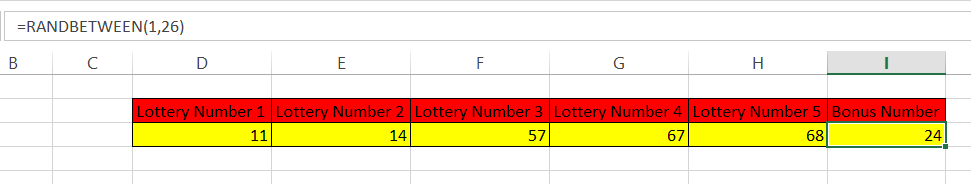Here is what the whole Lottery Number Generator looks like: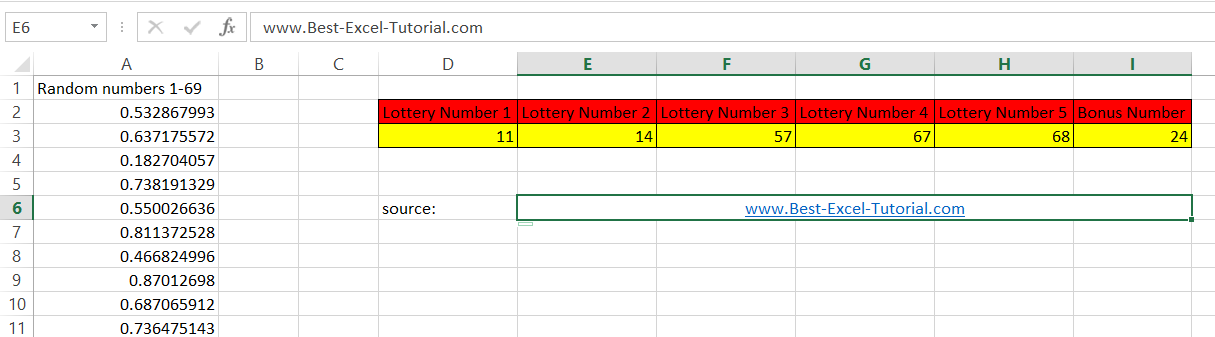Note: To make sure you get a new set of random numbers each time you recalculate the workbook, press “Ctrl + Alt + F9” to recalculate the workbook. Or you can set the calculation option to “Automatic” so that the workbook is recalculated automatically each time a change is made. To do this, go to “File” > “Options” > “Formulas” and select “Automatic” under “Calculation options.”

### How to generate one random number using RAND and ROUND functions?

An alternative method to generate a single random number between 1 and 26 in Microsoft Excel is to use the “RAND” function along with the “ROUND” function:

=ROUND(RAND()*25+1,0)

This formula generates a random decimal number between 0 and 1, multiplies it by 25 to scale it to the desired range (1 to 26), and then rounds the result to the nearest whole number using the “ROUND” function.

See also  How to count cells with a specific content in a range?

In conclusion, generating random numbers in Microsoft Excel can be done using a variety of methods. You can use the “RANDBETWEEN” function to generate a single random number within a specific range, or you can use a combination of the “RAND”, “ROUND”, “RANK”, “INDEX”, and “MATCH” functions to generate a list of unique random numbers. The choice of method will depend on your specific requirements and the type of random numbers you want to generate.

It’s important to keep in mind that Excel uses a pseudorandom number generator, so the numbers generated may not be truly random. To ensure that the numbers generated are as random as possible, it’s a good idea to recalculate the workbook periodically or set the calculation option to “Automatic.”

Of course, this spreadsheet is just a tool, and it is not a guarantee that you will win the main prize. Probability for that remains the same unfortunately.

We wish you generated only lucky lottery numbers and won a fortune with our random lottery number generator.

## How to Improve the Accuracy of Your Lottery Number Generator

The accuracy of your lottery number generator depends on a number of factors, including the number of random numbers generated, the range of numbers generated, and the method used to generate the random numbers.

To improve the accuracy of your lottery number generator, you can do the following:

• Generate a large number of random numbers. The more random numbers you generate, the more likely it is that you will generate a winning combination.
• Generate a wide range of random numbers. The wider the range of numbers generated, the more likely it is that you will generate a winning combination.
• Use a reliable method to generate random numbers. There are a number of methods for generating random numbers, and some methods are more reliable than others.

You can also improve the accuracy of your lottery number generator by using a combination of different methods. For example, you could use the RANDBETWEEN function to generate a random number between 1 and 69, and then use the RANK function to rank the numbers. This will give you a list of the 69 most random numbers, which you can then use to generate your lottery numbers.

It is important to note that no lottery number generator can guarantee that you will win the lottery. However, by following the tips above, you can improve your chances of winning.

Here are some additional tips for improving the accuracy of your lottery number generator:

• Use a random number generator that is certified by a reputable organization.
• Make sure that the random number generator is not biased.
• Test the random number generator to make sure that it is generating truly random numbers.

By following these tips, you can improve the accuracy of your lottery number generator and increase your chances of winning the lottery.

## Key Takeaways

• To generate random lottery numbers in Excel, you can use the RANDBETWEEN function.
• To generate random lottery numbers without repeats, you can use the RANDBETWEEN and MATCH functions.
• You can improve the accuracy of your lottery number generator by generating a large number of random numbers, generating a wide range of random numbers, and using a reliable method to generate the random numbers.

## FAQ

• Q: How many random numbers should I generate?
• A: The more random numbers you generate, the more likely it is that you will generate a winning combination. However, generating too many random numbers can be time-consuming. A good rule of thumb is to generate at least 100 random numbers.
• Q: What range of numbers should I generate?
• A: The range of numbers you generate should depend on the lottery you are playing. For example, if you are playing the Powerball lottery, you should generate numbers between 1 and 69.
• Q: What is the best method for generating random numbers?
• A: There is no one-size-fits-all answer to this question. The best method for generating random numbers will depend on your specific needs. However, some reliable methods include using the RANDBETWEEN function in Excel, using a random number generator from a reputable organization, or using a physical random number generator such as a dice or coin.